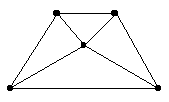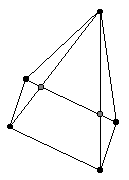## Project 14: Drawings of 3-Polytopes

If one draws a plane graph which represents a 3-polytope there is essentially only one way to do this, even though different drawings may look rather different. For example, in the Figure below two different drawings of a 4-gonal pyramid have been drawn.The number of faces with different numbers of sides for either of these two drawings is: p3 = 4 and p4 = 1. (pk denotes the number of faces with k sides.)

Now consider drawings of a 4-gonal pyramid drawn in a manner so as to suggest that the 3-dimensionality of the 3-polytope.

An examples of such a drawing is shown below:Note that this drawing has extra crossings which do not actually represent vertices of the drawings. However, suppose that we now add these "accidental crossings" as additional vertices. The result for the graph above is shown below:Let us call this procedure making of a graph from a drawing (MGD). We now get one or more face vectors different from the original one.

Problem

1. Is there any relation between the set of face vectors that arise from all the different possible drawings of a fixed 3-polytope by the procedure described above?

2. For a fixed number of of vertices, which 3-polytope gives the largest number of face vectors by this procedure?

3. For a fixed number of vertices, which 3-polytope admits some drawing with the largest number of vertices which have to be added using the MGD process?

Joseph Malkevitch
Department of Mathematics and Computing
York College (CUNY)
Jamaica, New York 11451-0001
email: malkevitch@york.cuny.edu
(Comments and results related to the project above are welcome.)

Acknowledgements
Some of this work was prepared with partial support from the National Science Foundation (Grant Number: DUE 9555401) to the Long Island Consortium for Interconnected Learning (administered by SUNY at Stony Brook, Alan Tucker, Project Director).